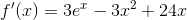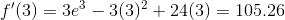# AP Calculus BC : Instantaneous Rate of Change, Average Rate of Change, and Linear Approximation

## Example Questions

### Example Question #1 : Instantaneous Rate Of Change, Average Rate Of Change, And Linear Approximation

Calculate the derivative of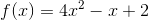at the point.Explanation:

There are 2 steps to solving this problem.

First, take the derivative of.

Then, replace the value of x with the given point.

For example, if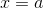, then we are looking for the value of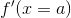, or the derivative ofat.Calculate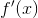Derivative rules that will be needed here:

• Derivative of a constant is 0. For example,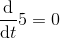• Taking a derivative on a term, or using the power rule, can be done by doing the following: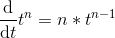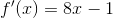Then, plug in the value of x and evaluate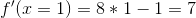### Example Question #2 : Instantaneous Rate Of Change, Average Rate Of Change, And Linear Approximation

Evaluate the first derivative if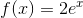and.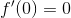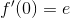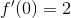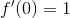Explanation:

First we must find the first derivative of the function.

Because the derivative of the exponential function is the exponential function itelf, or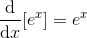and taking the derivative is a linear operation,

we have that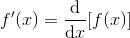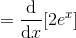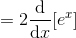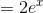Now setting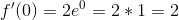Thus### Example Question #3 : Instantaneous Rate Of Change, Average Rate Of Change, And Linear Approximation

Find the rate of change of f(x) when x=3.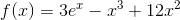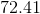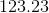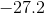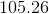Explanation:

Find the rate of change of f(x) when x=3.To find a rate of change, we need to find the derivative.

First, recall the following rules: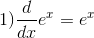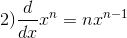We can apply these two derivative rules to our function to get  our first derivative. Then we need to plug in 3 for x and solve.